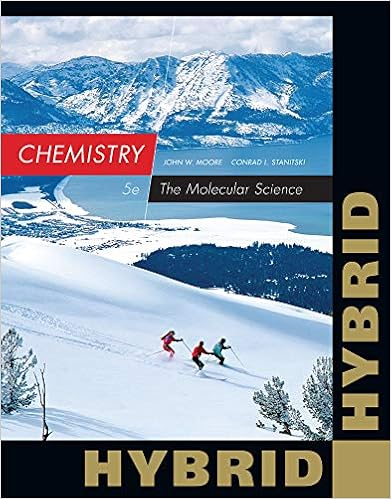# A flask has a mass of 7823 g when empty and 59363 g

• Test Prep
• 17
• 67% (3) 2 out of 3 people found this document helpful

This preview shows page 3 - 6 out of 17 pages.

##### We have textbook solutions for you!
The document you are viewing contains questions related to this textbook.The document you are viewing contains questions related to this textbook.
Chapter 2 / Exercise 2.1
Chemistry: The Molecular Science
Moore/StanitskiExpert Verified
5. A flask has a mass of 78.23 g when empty and 593.63 g when filled completely with water. When the same flask is filled with sulfuric acid, its mass is 1026.57 g. What is the density of sulfuric acid? (Assume water has a density of 1.000 g/mL) A. 1.992 g/mL B. 1.840 g/mL C. 1.729 g/mL D. 1.598 g/mL E. 0.543 g/mL Answer: B. 1.840 g/mL 593.63 g Mass of flask + water – 78.23 g Mass of flask 515.40 g Mass of water Use the density of water to find the volume occupied by 515.40 g of water: Volume (mL) = 221 mL HO515.40 g HO1.000 g HO= 515.40 mL
2
##### We have textbook solutions for you!
The document you are viewing contains questions related to this textbook.The document you are viewing contains questions related to this textbook.
Chapter 2 / Exercise 2.1
Chemistry: The Molecular Science
Moore/StanitskiExpert Verified
4Since the flask is filled completely with 515.40 mL of water, the volume of the flask is 515.40 mL. So the volume of sulfuric acid in the flask is also 515.40 mL. 1026.57 g Mass of flask + acid – 78.23 g Mass of flask 948.34 g Mass of acid Density = mass948.34 g= volume515.40 mL= 1.840 g/mL
6. A room is 4 meters wide by 6 meters long by 3 meters high and has an air conditioner that exchanges air at a rate of 1200 liters per minute. How long will it take for the air conditioner to exchange the air in the room once? (1 mL = 1 cm3)
7. Assume that the total volume of seawater on Earth is 1.55x1021liters and that the density of seawater is 1.03 g/mL. If seawater is 3.50 % by mass NaCl, what is the total mass of NaCl present in seawater, in units of kilograms?
24kg
kg
kg
58. How many protons, neutrons, and electrons are there in the anion 31P3–?
•••